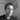# Closures Introduction

May 04, 2019

This post describes a common javascript interview topic - closures and scope. I've written this mostly for my own reference, but I hope it can be of some use to someone. Let me know if something should be clarified or corrected.

Closures are function behaviour which gives access to the surrounding environment. There are two main concepts required when talking about functions:

• execution context (where/when you RUN the function).
• lexical context (where you WRITE the function).

A key idea here is the way Javascript handles SCOPE, defined on MDN here as:

"The current context of execution. The context in which values and expressions are 'visible', or can be referenced. If a variable or other expression is not 'in the current scope,' then it is unavailable for use. Scopes can also be layered in a hierarchy, so that child scopes have access to parent scopes, but not vice versa."

So Javascript keeps track of what was available to the function when it was written. This allows some useful behaviour. ES6 also introduced some new ways to do variable declaration, with consequences for this behaviour.

## Let's get to the problem they'll show in an interview:

What is the expected output of this code?

``````const array = [1, 2, 3, 4];
for (var i = 0; i < array.length; i++) {
setTimeout(function() {
console.log("I am at index " + i);
}, 3000);
}``````

The naive expectation is for the output to be:

``````I am at index 1
I am at index 2
I am at index 3
I am at index 4``````

but it's actually:

``````I am at index 4
I am at index 4
I am at index 4
I am at index 4``````

## Why?

The key to understanding this behaviour is scope. In the above script, `i` is "functionally scoped", because it was declared using `var`. This means that it is declared once, and then overwritten with each loop. This process is completed (reaching 4) before the `setTimeout` returns. Things might seem a little murky here, as the order of execution depends on the callstack and the callback queue. The idea is that the stack will be completed before the callback queue, and because the setTimeout is part of the webAPI, that is handled separately from the callback queue and its result is therefore returned after the stack is empty.

Lost?

If that is all new and/or jargon to you, I highly recommend this beginner-friendly intro video:

## So how can we fix the code?

Assuming you correctly identify the problem with the code, you'd then be asked how to correct it.

``````const array = [1, 2, 3, 4];
for (let i = 0; i < array.length; i++) {
setTimeout(function() {
console.log("I am at index " + i);
}, 3000);
}``````

Spot the difference? I've replaced `var` with `let`. What's the output?

``````I am at index 0
I am at index 1
I am at index 2
I am at index 3``````

Ok, so we're iterating over the indexes, but not the actual contents. How do we get those? Like this:

``````const array = [1, 2, 3, 4];
for (let i = 0; i < array.length; i++) {
setTimeout(function() {
console.log("I am at index " + array[i]); //difference is here.
}, 3000);
}``````

Fixed output:

``````I am at index 1
I am at index 2
I am at index 3
I am at index 4``````

# Why, though?

Because `let` is block scoped. This means that it's effectively stuck within the loop (at least in our example). There is a scope for each `i`. So we get a different `i` for each run through the loop, and therefore the `1,2,3,4` that we want. When, originally, we declared with `var`, that was in global scope and was just one variable.

If you want to read more on block vs function scope, the best resource I know is "You Don't Know Javascript", which you can read online for free. Here is the relevant chapter.

## IIFE and closures, finally

Avoiding `let`, there's another way to do this, which takes advantage of iffe (immediately invoked function expressions) and closures.

If we wrap our `setTimeout` in a function, that creates a closure- so the function has access to the variables in the containing environment.

``````const array = [1, 2, 3, 4];
for (var i = 0; i < array.length; i++) {
(function(exampleClosureVar) {
setTimeout(function() {
console.log("I am at index " + array[exampleClosureVar]);
}, 3000);
})(i);
}``````

The lexical scope is defined, as the name suggests, when the function is written, so our anonymous function has access to the var `i`, because it is "remembered" as being part of the function's scope. This will remain associated with the function (any function), so hopefully you can see how this can be useful for giving functions access to information/variables which they're not supposed to expose or directly change.

Here's a more detailed guide to closures.

As I said at the start, I hope this can be of some use to someone. Let me know if something should be clarified or corrected.